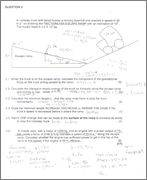# Work,Energy And Power, Please Check My Work

• TheRedDevil18
;)hehe, yeah that's true. you have to keep as many decimal places as possible until the end, which is pretty annoying. you could use fractions as you go through the calculation, but that is maybe even more annoying!

## Homework Statement## The Attempt at a Solution

5.1) fg// = mgSin(theta)
= 30437.12 N

5.2) Ek = 1/2*m*v^2
= 1/2*(1.2*10^4)*36^2
= 7776000 J

5.3) Wnet = Ek
7776000 = 30437.12*delta x
delta x = 255.48 m

5.4) Decrease

5.5) Make the surface rough to increase friction

Fnet = ma
3195.9 = 1260*a
a = -2.54m.s^-2

vf = vi+a*t
0 (At the top of the ramp) = 20 (Is this the initial velocity?, not sure) + (-2.54)*t
t = 7.87 s

P = W/t
65700 (90% efficient) = W/7.87
W = 517059 J

Wnet = Fnet*delta x
517059 = 3195.9*delta x
delta x = 161.79 m

Therefore, No, as the length of the ramp is 255.48m, the car will only make 161.79m

you've got them all right except question 5.6 (and question 5.6 is a little difficult, just because it is a bit difficult to see what they are asking). They say the car is at a constant velocity as it moves along the ramp. This means there is no net force! They give you the force the engine must produce (3159.9N) But you could have worked this out yourself if you wanted to. Anyway, what the question is asking, is to calculate the power the engine is producing in this situation. And then to see if this power is less than the max possible 'power output' for that engine.

BruceW said:
you've got them all right except question 5.6 (and question 5.6 is a little difficult, just because it is a bit difficult to see what they are asking). They say the car is at a constant velocity as it moves along the ramp. This means there is no net force! They give you the force the engine must produce (3159.9N) But you could have worked this out yourself if you wanted to. Anyway, what the question is asking, is to calculate the power the engine is producing in this situation. And then to see if this power is less than the max possible 'power output' for that engine.

Yeah, I know as I also got confused with what they are asking so I thought 3159.9 N was the net force. So here's what I came up with

So if power = force*velocity, then
p = 3195.9*20
= 63918 W
= 63.92 kw

And if the car is 90% effiecient
90%*73
= 65.7 kw

So yes the car will make it up the hill as the power required is lower than the power given out by the engine.

Correct?

exactly! nice work :)

edit: also, you might need to think a bit more about how many significant figures you use for your answers. I'm guessing you are using g=9.8m/s^2, so for example in question 5.1 you give an answer of 30437.12 N so it looks like you are suggesting you have an accuracy of plus or minus 0.01 N when really you have an accuracy more like plus or minus 100 N But anyway, this depends on how strict the teacher is I guess. He/She may not mind about the number of significant figures you give.

Last edited:
edit: also, you might need to think a bit more about how many significant figures you use for your answers. I'm guessing you are using g=9.8m/s^2, so for example in question 5.1 you give an answer of 30437.12 N so it looks like you are suggesting you have an accuracy of plus or minus 0.01 N when really you have an accuracy more like plus or minus 100 N But anyway, this depends on how strict the teacher is I guess. He/She may not mind about the number of significant figures you give.

Thanks Bruce, yes the teachers are pretty strict with rounding off. It is stated that we should round off to 2 decimal places otherwise they deduct a markah, that's not too bad, at least they tell you in advance how many decimal places they want :)

BruceW said:
ah, that's not too bad, at least they tell you in advance how many decimal places they want :)

Yeah, but it can get pretty annoying especially when you round off early in the question which causes your final answer to go offhehe, yeah that's true. you have to keep as many decimal places as possible until the end, which is pretty annoying. you could use fractions as you go through the calculation, but that is maybe even more annoying!